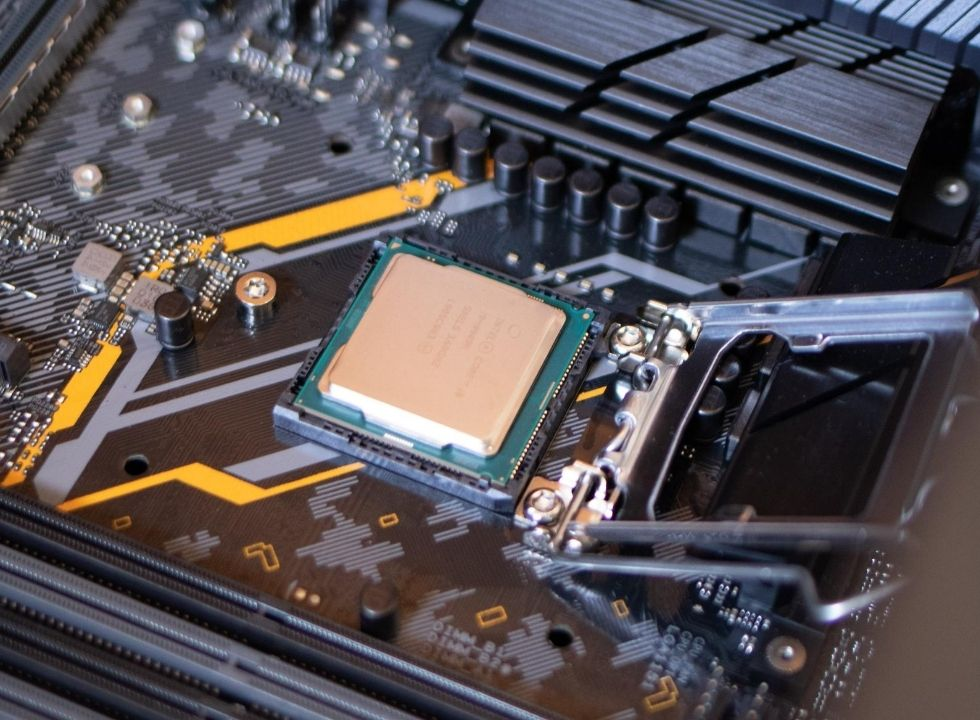What is CPU and how it works

Computer chip means CPU or processor, the full form of which is the Central Processing Unit which is called the brain of a computer. The CPU does all the processing of the computer.

## To understand how this chip works, let's first look at what is inside a computer chip?

At the core of a chip is a transistor. A transistor is a type of electrical switch. A chip contains millions upon millions of transistors. Each transistor can have two states, on and off. A computer that does anything has a binary number system at the root of everything. The binary number system is a number system where only 1 and 0 are these two numbers. The reason for using binary is that the transistor can only have two states. The on-state of a transistor is expressed by K1 and the off state by 0.

## How does a CPU work?

The transistors are on or off according to the binary sequence for each of our commands. Each transistor can hold one bit. The bit is every digit of binary. Any operation of the computer is taken to binary then the computer chip understands them and gives the output by processing.

When we do some work on the computer, suppose I type A from the keyboard but the computer does not understand A. The value of A in binary 01000001, some computer programs decode A and send this 8-bit binary number to the processor. The 8 transistors in the processor are then on or off according to the sequence of binary bits. Again, even if we do some calculations, the decimal 10 based numbers are converted to binary, then the computer chip calculates and sends the output to the binary, but before it is displayed on the computer monitor, some programs convert it to the decimal system and display the results. A simple calculator works in the same way.

Different computer programs or software are written in different types of programming languages. Write is compiled with the help of a compiler and taken to binary and sent to the chip as an instruction set. With the help of millions of transistors in the chip, they are processed and output comes to us.

A strange thing is that any work on our computer is done with the help of mathematics. All we do is go to a level and become binary then a chip works through the mathematical operation between the binary bits.
What is CPU and how it works?Reviewed by Shariful Islam Shobuj on August 01, 2020 Rating: 5